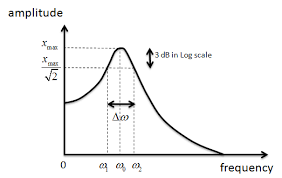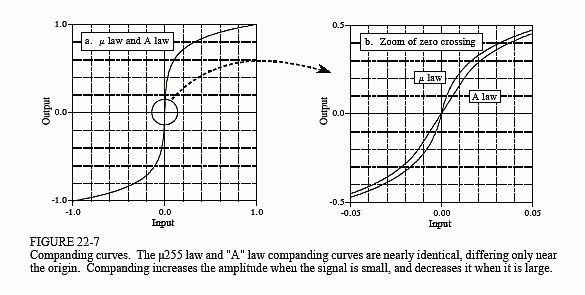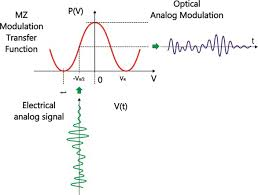#### Howdy, Stranger!

It looks like you're new here. If you want to get involved, click one of these buttons!

Options

# Negative Entropy

edited March 29 in General

I'd like to start a discussion about negative entropy. Consider the idea of the arc of history bending to a positive resolution.

Alexander the Great conquered large portions of Europe and Asia. At the time, being conquered was a disaster, but centuries later it provided languages with a unified basis. So negative entropy has a component of duration. This can be seen as moving to a global optima.

I am interested in the levels of the Ackermann function for modeling non-local optima.

Do folks have other examples demonstrating negative entropy and it's principles?

## Comments

• Options
1.

Negative entropy is a measure of order and I apply it quite frequently.

Comment Source:Negative entropy is a measure of order and I apply it quite frequently.
• Options
2.
edited April 12

Proposed Principles

Negative entropy is more complex than positive entropy.

I submit that Maslow's hierarchy of needs is a good model of negative entropy, particularly his later models. See https://www.simplypsychology.org/maslow.html . Note the anthropomorphic aspect.

The Category Theory Zulip group discusses music and ascetics as an unavoidable human trait.

Comment Source:Proposed Principles Negative entropy is more complex than positive entropy. I submit that Maslow's hierarchy of needs is a good model of negative entropy, particularly his later models. See https://www.simplypsychology.org/maslow.html . Note the anthropomorphic aspect. The Category Theory Zulip group discusses music and ascetics as an unavoidable human trait. 
• Options
3.

I can't help feeling that the concept of negative entropy is central to the betterment of mankind.

Comment Source:I can't help feeling that the concept of negative entropy is central to the betterment of mankind.
• Options
4.

Negative entropy is just entropy with a reversed sign and so is a measure of order, or of lower complexity. I have some good recent examples but this paper I wrote using Shannon entropy lays out some practical applications https://www.intechopen.com/books/applications-of-digital-signal-processing/entropic-complexity-measured-in-context-switching

Comment Source:Negative entropy is just entropy with a reversed sign and so is a measure of order, or of lower complexity. I have some good recent examples but this paper I wrote using Shannon entropy lays out some practical applications https://www.intechopen.com/books/applications-of-digital-signal-processing/entropic-complexity-measured-in-context-switching 
• Options
5.

Here's the way to think about using negative entropy to find an optimal solution. Consider autonomous vs non-autonomous differential equations. One way to think about the distinction is that the transfer function for non-autonomous only depends on the presenting input. Thus, it acts like an op-amp with infinite bandwidth. Or below saturation it gives perfectly linear amplificationIn contrast, for an autonomous formulation, the amplification depends on prior values so it requires a time-domain convolution or a frequency-domain transfer functionYet there are many other non-autonomous formulations that aren't linear, for example a companding transfer that takes the square root of the input (used for compressing the dynamic range of a signal).What does this have to do with entropy? Well that transfer function can get very strange but still possess underlying order. Yet that order or pattern may be difficult to discern without adequate information. So consider if the non-autonomous transfer function itself is something odd, such as an unknown and potentially complex sinusoidal modulation. This occurs in Mach-Zehnder modulation. The effect is to distort the input enough to fold the amplitude at certain points.The difficulty is if we have little knowledge of the input forcing or the modulation, we will not be able to decode anything. But with a measure such as Negative Shannon Entropy, we can see how far we can go with limited info.

So consider this output waveform that we are told is due to Mach-Zehnder modulation of an unknown inputAll we know is that there may be a basis forcing that consists of a couple of sinusoids, and that there is an obvious non-autonomous complex modulation that is generating the above waveform

The idea is that we test out various combinations of sinusoidal parameters and then maximize the Shannon entropy of the power spectrum of the transfer from input to output (see the citation in the previous post). We can do this calculating a discrete Fourier transform or an FFT and multiplying by the complex conjugate to get the power spectrum. For a perfectly linear amplification as in the first example, it is essentially a delta function at a frequency of zero, indicating maximum order with a maximum negative Shannon entropy. And for a single sinusoidal frequency modulation, the power spectrum would be a delta shifted to the frequency of the modulation. Again this will be a maximally-ordered amplification, and again with a maximum in negative Shannon entropy. Yet, in practical terms, perhaps something such as a Renyi or Tsallis entropy measure would work even better than Shannon entropy. Actually, the Tsallis entropy is close to describing a mean-square variance error in a signal, whereby it exaggerates clusters or strong excursions when compared against a constant background.

So this is what I have used that works quite well. I essentially maximize the normalized mean-squared variance of the power spectrum

$$\frac{\sum (F(\omega)-<F(\omega)>)^2}{\sum F(\omega)}$$ The result of a search algorithm of input sinusoidal factors to maximize the power spectrum variance value is this power spectrumwhich stems from this optimal input forcingNote that this is not the transfer modulation, which we still need to extract from the power spectrum.

As a result, this negative entropy algorithm is able to deconstruct or decode a Mach-Zehnder modulation of two sinusoidal factors that's encoding an input forcing of another pair of sinusoidal factors. So essentially we are able to find 4 unknown factors (or 8 if both amplitude and phase are included) by only searching on 2 factors (or 4 if amplitude and phase are included). But how is that possible? It's actually not a free lunch because the power spectrum calculation is essentially testing all possible modulations in parallel and the negative entropy calculation is keeping track of the frequency components that maximize the delta functions in the spectrum. That is the mean-square variance is weighting greater excursions than a flat highly-random background would.

From the paper, this is the general idea. For negative entropy we are looking for the upper spectrum, not the lower, which is a maximum entropyGood luck, this works well for certain applications. It may even work better in a search algorithm than if you did a pure RMS minimization of fitting the 4 sinusoidal factors directly against the output, as it may not fall into local minima as easily. Doing the power spectrum helps to immediately broaden the search I think.

Comment Source:Here's the way to think about using negative entropy to find an optimal solution. Consider autonomous vs non-autonomous differential equations. One way to think about the distinction is that the transfer function for non-autonomous only depends on the presenting input. Thus, it acts like an op-amp with infinite bandwidth. Or below saturation it gives perfectly linear amplification ![](https://pbs.twimg.com/media/EyUEt_2U8AIhkhg.png) In contrast, for an autonomous formulation, the amplification depends on prior values so it requires a time-domain convolution or a frequency-domain transfer function ![](https://pbs.twimg.com/media/EyUGgCeU8AEeg0r.png) Yet there are many other non-autonomous formulations that aren't linear, for example a companding transfer that takes the square root of the input (used for compressing the dynamic range of a signal). ![](https://pbs.twimg.com/media/EyUHXfVVcAEvEkV.png) What does this have to do with entropy? Well that transfer function can get very strange but still possess underlying order. Yet that order or pattern may be difficult to discern without adequate information. So consider if the non-autonomous transfer function itself is something odd, such as an unknown and potentially complex sinusoidal modulation. This occurs in Mach-Zehnder modulation. The effect is to distort the input enough to fold the amplitude at certain points. ![](https://pbs.twimg.com/media/EyUIsZRVIAUcfYY.png) The difficulty is if we have little knowledge of the input forcing or the modulation, we will not be able to decode anything. But with a measure such as Negative Shannon Entropy, we can see how far we can go with limited info. So consider this output waveform that we are told is due to Mach-Zehnder modulation of an unknown input ![](https://imagizer.imageshack.com/img922/3888/lLoVr3.png) All we know is that there may be a basis forcing that consists of a couple of sinusoids, and that there is an obvious non-autonomous complex modulation that is generating the above waveform The idea is that we test out various combinations of sinusoidal parameters and then maximize the Shannon entropy of the *power spectrum* of the transfer from input to output (see the citation in the previous post). We can do this calculating a discrete Fourier transform or an FFT and multiplying by the complex conjugate to get the power spectrum. For a perfectly linear amplification as in the first example, it is essentially a delta function at a frequency of zero, indicating maximum order with a maximum negative Shannon entropy. And for a single sinusoidal frequency modulation, the power spectrum would be a delta *shifted* to the frequency of the modulation. Again this will be a maximally-ordered amplification, and again with a maximum in negative Shannon entropy. Yet, in practical terms, perhaps something such as a Renyi or Tsallis entropy measure would work even better than Shannon entropy. Actually, the [Tsallis entropy](https://en.wikipedia.org/wiki/Tsallis_entropy) is close to describing a mean-square variance error in a signal, whereby it exaggerates clusters or strong excursions when compared against a constant background. So this is what I have used that works quite well. I essentially maximize the normalized mean-squared variance of the power spectrum $$\frac{\sum (F(\omega)-<F(\omega)\>)^2}{\sum F(\omega)}$$ The result of a search algorithm of input sinusoidal factors to maximize the power spectrum variance value is this power spectrum ![](https://imagizer.imageshack.com/img924/5228/w54jkW.png) which stems from this optimal input forcing ![](https://imagizer.imageshack.com/img923/3659/wE7Gon.png) Note that this is not the transfer modulation, which we still need to extract from the power spectrum. As a result, this negative entropy algorithm is able to deconstruct or decode a Mach-Zehnder modulation of two sinusoidal factors that's encoding an input forcing of another pair of sinusoidal factors. So essentially we are able to find 4 unknown factors (or 8 if both amplitude and phase are included) by only searching on 2 factors (or 4 if amplitude and phase are included). But how is that possible? It's actually not a free lunch because the power spectrum calculation is essentially testing all possible modulations in parallel and the negative entropy calculation is keeping track of the frequency components that maximize the delta functions in the spectrum. That is the mean-square variance is weighting greater excursions than a flat highly-random background would. From the paper, this is the general idea. For negative entropy we are looking for the upper spectrum, not the lower, which is a maximum entropy ![](https://imagizer.imageshack.com/img922/6891/XKauf7.png) Good luck, this works well for certain applications. It may even work better in a search algorithm than if you did a pure RMS minimization of fitting the 4 sinusoidal factors directly against the output, as it may not fall into local minima as easily. Doing the power spectrum helps to immediately broaden the search I think. 
• Options
6.

Wonderful response Paul. I'll work on mastering your material and then reply.

Comment Source:Wonderful response Paul. I'll work on mastering your material and then reply.
• Options
7.

In 1979 I rolled into Coco Beach, Florida as a new Air Force recruit. I would work at AFTAC which monitors nuclear events. I was assigned to TGS, the geophysical division, as a seismologist.

It was the end of one era and the beginning of another. We had access to interactive signal processing software that would have made Stephen Wolfram proud. Spectrums, cepstrums, convolution filters and so on. Unfortunately, we were constrained to the realm of statistics and statistical sigmas. From what I understand, we still are.

Comment Source:In 1979 I rolled into Coco Beach, Florida as a new Air Force recruit. I would work at AFTAC which monitors nuclear events. I was assigned to TGS, the geophysical division, as a seismologist. It was the end of one era and the beginning of another. We had access to interactive signal processing software that would have made Stephen Wolfram proud. Spectrums, cepstrums, convolution filters and so on. Unfortunately, we were constrained to the realm of statistics and statistical sigmas. From what I understand, we still are. 
• Options
8.
edited April 16

Daniel, if you haven't come across it you might enjoy Brillouin's 1956 originating text "Science and Information Theory": https://www.informationphilosopher.com/solutions/scientists/brillouin/

Comment Source:Daniel, if you haven't come across it you might enjoy Brillouin's 1956 originating text "Science and Information Theory": https://www.informationphilosopher.com/solutions/scientists/brillouin/
• Options
9.

Regarding Laplace and his demon and the possibility of determinism, these items from the past week:

Comment Source:Regarding Laplace and his demon and the possibility of determinism, these items from the past week: 1. [On the Shoulders of Laplace](https://www.sciencedirect.com/science/article/pii/S0031920121000510) 1. [BBC podcast on Laplace](https://podcasts.google.com/feed/aHR0cDovL3d3dy5yc3NtaXguY29tL3UvODMyMjkzNi9yc3MueG1s/episode/dXJuOmJiYzpwb2RjYXN0Om0wMDB0d2dq?ep=14) 
• Options
10.
edited April 18

Paul, I'm not getting the connection between determinism and negative entropy although I'm happy to discuss almost any subject. I will say in my mind that Laplace's equation and Hamilton's equation are two of the most beautiful constructs in mathematics and physics. My own research delves into dynamics or so called chaos theory. I'd love to try and put something together on the link between dynamics and negative entropy.

Comment Source:Paul, I'm not getting the connection between determinism and negative entropy although I'm happy to discuss almost any subject. I will say in my mind that Laplace's equation and Hamilton's equation are two of the most beautiful constructs in mathematics and physics. My own research delves into dynamics or so called chaos theory. I'd love to try and put something together on the link between dynamics and negative entropy. 
• Options
11.

If determinism follows an ordered pattern then that will show up as a higher value of negative entropy. That's all there is to it, which is shown in the last pic I posted.

Comment Source:If determinism follows an ordered pattern then that will show up as a higher value of negative entropy. That's all there is to it, which is shown in the last pic I posted. 
• Options
12.

Oops, I should have got that myself. OK, determinism and negative entropy are connected.

Comment Source:Oops, I should have got that myself. OK, determinism and negative entropy are connected.
Sign In or Register to comment.